Courses

# Test: Strain Energy

## 10 Questions MCQ Test Topicwise Question Bank for GATE Civil Engineering | Test: Strain Energy

Description
This mock test of Test: Strain Energy for Civil Engineering (CE) helps you for every Civil Engineering (CE) entrance exam. This contains 10 Multiple Choice Questions for Civil Engineering (CE) Test: Strain Energy (mcq) to study with solutions a complete question bank. The solved questions answers in this Test: Strain Energy quiz give you a good mix of easy questions and tough questions. Civil Engineering (CE) students definitely take this Test: Strain Energy exercise for a better result in the exam. You can find other Test: Strain Energy extra questions, long questions & short questions for Civil Engineering (CE) on EduRev as well by searching above.
QUESTION: 1

### Total strain energy stored in a simply supported beam of span ‘L' and flexural rigidity ‘El’ subjected to a concentrated load ‘W at the centre is equal to

Solution: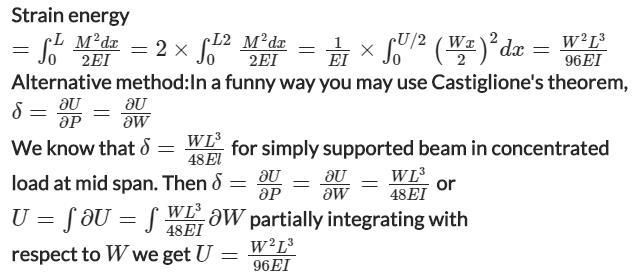QUESTION: 2

### Which one of the following gives the correct expression for strain energy stored in a beam of length L and of uniform cross-section having moment of inertia I and subjected to constant bending moment M?

Solution: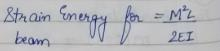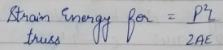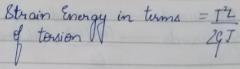QUESTION: 3

### A square bar of side 40 mm and length 1 m is subjected to an axial load P. The same bar is then used as a cantilever beam and  subjected to an end load P. The ratio of the strain energies, stored in the bar in the second case to that stored in the first case, is

Solution: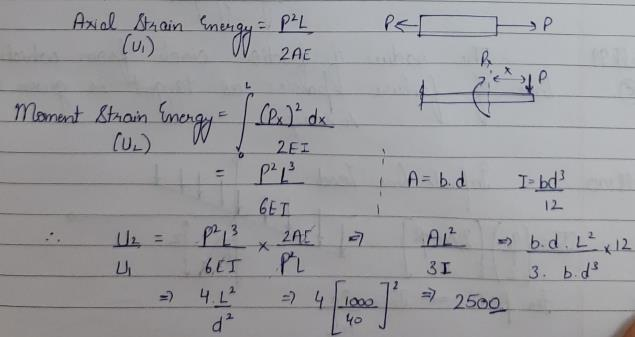QUESTION: 4

A bar of length L and of uniform cross-sectional area A. and second moment of area I is subjected to a pull P. If young’s modulus of elasticity of the bar material is E, the expression for strain energy stored in the bar will be

Solution:
QUESTION: 5

Strain energy stored in a body of volume V subjected to uniform stress σ is

Solution: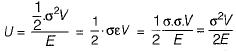QUESTION: 6

A bar having length L and uniform cross-section with area A is subjected to both tensile force P and torque T. If G is the shear modulus and E is the Young’s modulus, the internal strain energy stored in the bar is

Solution:

Total strain energy,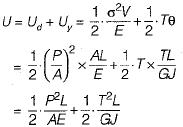QUESTION: 7

What is the strain energy stored in a body volume V with stress σ due to gradually applied load?

Solution:

U = σ2V/2E

QUESTION: 8

Strain energy in a member is

Solution:
QUESTION: 9

For the state of stress of pure shear τ, the strain energy stored per unit volume in the elastic, homogeneous isotropic material having elastic constants E and v will be

Solution:
QUESTION: 10

A beam of length L, simply supported at ends, carries a load W at a distance of a from one end and at a distance of b from other end such that a + b = L. If El is flexural rigidity of the beam, how much strain energy is absorbed in beam?

Solution: